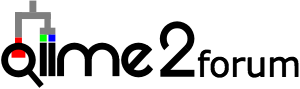# Explanation of Songbird differentials (Reference, Differentials) in regards to --p-formula

I have a question regarding my formula for songbird, which is in the following format

–p-formula “Treatment+DPT+Parasites”

In the Treatment column of my metadata file, I have 4 categories (Treatment groups), C (control), MeOH, F9, and F150. However, the C category is missing from the differentials. I understand that the number of variables reported for the Treatment column should be K-1 or 3 which explains why the C category is missing. I just want to confirm that the C category is the reference? AndTreatment_DPT_Parasites_differentials_pdp02_200k-viz.qzv (1.2 MB) Treatment_DPT_Parasite_qurro_plot_q2.qzv (857.2 KB) , that the intercept is the mean abundance of the microbes in the C category and that the differential values in the MeOH, F9 and F150 columns are the log-fold changes of the microbes compared to the C (reference) category?

@Johnny_Sena, correct, the C category here is the reference here.

You are thinking about the interpret correctly. You can think of the linear regression coefficients as follows

``````(mean C) + (mean MeOH - mean C)  + (mean F9- mean C) + (mean F150 - mean C)
``````

So your intercept is literally the mean proportions of your C category in log coordinates. And all of the other coefficients are differences between the means in log coordinates. We call these differentials because they are literally per-microbe differences between two groups.

2 Likes